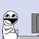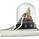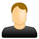# Indicators: Better Volume Indicator & InstrumentVolume

62575 visualizzazioni
Better Volume Indicator
-----------------------------------------
This is a direct port of a famous indicator from Tradestation platform.

BVI improves on your typical volume histogram by coloring the bars based on 5 criteria:
* Volume Climax Up – high volume , high range, up bars (red)
* Volume Climax Down – high volume , high range, down bars (white)
* High Volume Churn – high volume , low range bars (green, barcolor= blue)
* Low Volume – low volume bars (yellow)
* Volume Climax plus High Volume Churn – both the above conditions (magenta)

When there are no volume signals the default histogram bar coloring is cyan.

Bars can also be colored to match volume color. Enable "Change BarColors?" in the options page.

Volume Climax Up bars are typically seen at:
* The start of up trends
* The end of up trends, and
* Pullbacks during down trends.

Volume Climax Down bars are typically seen at:
* The start of down trends
* The end of down trends, and
* Pullbacks during up trends.

High Volume Churn bars are typically seen at:
* The end of up trends
* The end of down trends, and
* Profit taking mid-trend.

Low Volume bars are typically seen at:
* The end of up trends
* The end of down trends, and
* Pullbacks mid-trend.

http://emini-watch.com/free-stuff/volume...

Instrument Volume
-----------------------------------------
This is a simple script that allows you to plot volume for any instrument.

Very handy when you want to compare volumes. Just add multiple instances and select the symbol you want via Options page.

This script also gets close/open for the selected symbol. If you are itching to get started on Pinescripting (scripting language used at TV), I suggest trying out the following, using this script as the template:
- Show RSI for any instrument
(hint: "close" for the selected symbol is already in script. Do a "plot( rsi (c, 14))")
- MACD / CCI / ....
- Plot the difference (not correlation). This may be of interest in some instruments.
For ex. BTC in BTCE exchange mostly lags BITSTAMP .

Hope this piques your interest in Pine. Feel free to post in the Pinescript room if you have any queries.
```//
// @author LazyBear
//
// If you use this code in its original/modified form, do drop me a note.
//
study(title = "Better Volume Indicator [LazyBear]", shorttitle="BVI_LB")

//
// Configurable params
//
length=input(8, title="Lookback")
enableBarColors=input(false, type=bool)
use2Bars=input(true, type=bool)
lowVol=input(true, type=bool)
climaxUp=input(true, type=bool)
climaxDown=input(true, type=bool)
churn=input(true, type=bool)
climaxChurn=input(true, type=bool)

//
// Tweak the colors
//
lowVolColor = yellow
climaxUpColor = red
climaxDownColor = white
churnColor = green
climaxChurnColor = #8B008B
defColor = #00FFFF

//
// Don't change anything below this
//
range=tr
v=volume
v1 = close >= open ? (v * ((range) / ((2+(range*range)/10) * range + (open - close)))) : (v * (((range + close - open)) / (2+(range*range)/10) * range + (close - open)))
v2 = v - v1
v3 = v1 + v2
v4 = v1 * range
v5 = (v1 - v2) * range
v6 = v2 * range
v7 = (v2 - v1) * range
v8 = (range != 0 ?  v1 / range : 1)
v9 = (range != 0 ? (v1 - v2) / range : 1)
v10 = (range != 0 ?  v2 / range : 1)
v11 = (range != 0 ?  (v2 - v1) / range :  1)
v12 = (range != 0 ?  v3 / range : 1)
v13 = use2Bars ? v3 + v3 : 1
v14 = (use2Bars ? (v1 + v1)*(highest(high,2)-lowest(low,2)) : 1)
v15 = (use2Bars ? (v1 + v1-v2-v2)*(highest(high,2)-lowest(low,2)) : 1)
v16 = (use2Bars ? (v2 + v2)*(highest(high,2)-lowest(low,2)) : 1)
v17 = (use2Bars ? (v2 + v2-v1-v1)*(highest(high,2)-lowest(low,2)) : 1)
v18 =  ((use2Bars and (highest(high,2)!=lowest(low,2))) ? (v1+v1)/(highest(high,2)-lowest(low,2)) : 1)
v19 = ((use2Bars and (highest(high,2)!=lowest(low,2))) ? (v1+v1-v2-v2)/(highest(high,2)-lowest(low,2)) : 1)
v20 = ((use2Bars and (highest(high,2)!=lowest(low,2))) ? (v2+v2)/(highest(high,2)-lowest(low,2)) : 1)
v21 = ((use2Bars and (highest(high,2)!=lowest(low,2))) ? (v2+v2-v1-v1)/(highest(high,2)-lowest(low,2)) : 1)
v22 = ((use2Bars and (highest(high,2)!=lowest(low,2))) ? v13/(highest(high,2)-lowest(low,2)) : 1)

c1 = (v3 == lowest(v3, length) ?  1 : 0)
c2 = ((v4 == highest(v4, length) and close > open) ? 1 : 0)
c3 = ((v5 == highest(v5, length) and close > open) ? 1 : 0)
c4 = ((v6 == highest(v6, length) and close < open) ? 1 : 0)
c5 = ((v7 == highest(v7, length) and close < open) ? 1 : 0)
c6 = ((v8 == lowest(v8, length) and close < open) ? 1 : 0)
c7 = ((v9 == lowest(v9, length) and close < open) ? 1 : 0)
c8 = ((v10 == lowest(v10, length) and close > open) ? 1 : 0)
c9 = ((v11 == lowest(v11, length) and close > open) ? 1 :  0)
c10 = (v12 == highest(v12, length) ? 1 : 0)
c11 = (use2Bars and (v13==lowest(v13,length) and close > open and close > open) ? 1 : 0)
c12 = (use2Bars and (v14==highest(v14,length) and close > open and close > open) ? 1 : 0)
c13 = (use2Bars and (v15==highest(v15,length) and close > open and close < open) ? 1 : 0)
c14 = (use2Bars and (v16==lowest(v16,length) and close < open and close < open) ? 1 : 0)
c15 = (use2Bars and (v17==lowest(v17,length) and close < open and close < open) ? 1 : 0)
c16 = (use2Bars and (v18==lowest(v18,length) and close < open and close < open) ? 1 : 0)
c17 = (use2Bars and (v19==lowest(v19,length) and close > open and close < open) ? 1 : 0)
c18 = (use2Bars and (v20==lowest(v20,length) and close > open and close > open) ? 1 : 0)
c19 = (use2Bars and (v21==lowest(v21,length) and close > open and close > open) ? 1 : 0)
c20 = (use2Bars and (v22==lowest(v22,length)) ? 1 : 0)

c0=(climaxUp and (c2 or c3 or c8 or c9 or c12 or c13 or c18 or c19)) ? climaxUpColor : ((climaxDown and (c4 or c5 or c6 or c7 or c14 or c15 or c16 or c17)) ? climaxDownColor : ((churn and c10 or c20) ? churnColor : defColor))
v_color=(climaxChurn and (c10 or c20)) and (c2 or c3 or c4 or c5 or c6 or c7 or c8 or c9) ? climaxChurnColor : ((lowVol and (c1 or c11)) ? lowVolColor : c0)
plot(not enableBarColors ? volume : na, style=columns, linewidth=1, color = v_color)
plot(not enableBarColors ? sma(volume, length) : na, color=orange, linewidth=2)
barcolor(enableBarColors ? v_color : na)```
List of my free indicators: http://bit.ly/1LQaPK8
List of my indicators at Appstore: http://blog.tradingview.com/?p=970

## CommentiIn this chart, I turned the candle outlines & tails charcoal, . I also turned on the Priceline which changes color based on the newest candle. For what it's worth.
Rispondigrahvity
perfect...looks great @grahvity. Thanks.
RispondiAwesome - I'm quickly becoming a fan of your work and wanted to say thanks for sharing.

One question:
Using default input settings, I'm seeing green bars being painted (also in histogram) when it's a large range bar with high volume. In your description, it says it's a high churn bar with a low range bar.

Link to example on Bitmex XBTYUSD contract 2Hr chart.

Thoughts? and thanks again.
Risponditapehound
@tapehound, Friend, how did you do to color the bars and keep the histogram colored at the same time in tradingView?
Rispondi